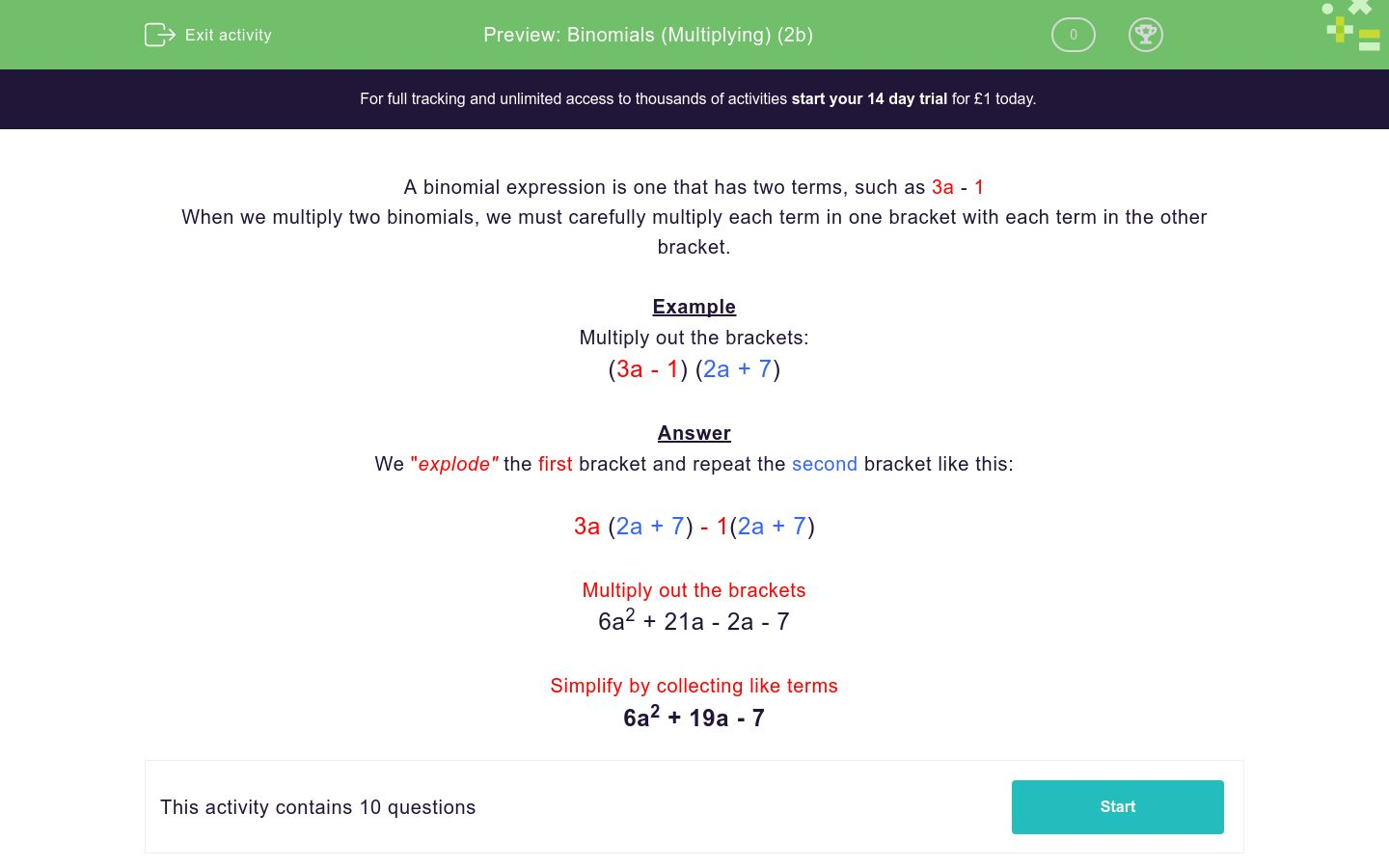# Binomials (Multiplying) (2b)

In this worksheet, students multiply together two binomial expressions.Key stage:  KS 4

Curriculum topic:  Algebra

Difficulty level:### QUESTION 1 of 10

A binomial expression is one that has two terms, such as 3a - 1

When we multiply two binomials, we must carefully multiply each term in one bracket with each term in the other bracket.

Example

Multiply out the brackets:

(3a - 1) (2a + 7)

Answer

We "explode" the first bracket and repeat the second bracket like this:

3a (2a + 7) - 1(2a + 7)

Multiply out the brackets

6a2 + 21a - 2a - 7

Simplify by collecting like terms

6a2 + 19a - 7

Multiply out the brackets:

(3a + 2) (2a + 1)

a2+7a +1

3a2+5a +2

3a2+3a +1

6a2+7a +2

Multiply out the brackets:

(2a + 2) (2a + 3)

4a2+2a +6

4a2+10a +6

4a2+8a +6

4a2+6a +5

Multiply out the brackets:

(2a + 6) (2a + 1)

a2+14a +6

4a2+14a +7

2a2+14a +7

4a2+14a +6

Multiply out the brackets:

(3a + 4) (2a - 1)

3a2+a -4

6a2+5a -4

6a2+a -4

3a2+4a +3

Multiply out the brackets:

(2a + 3) (3a - 2)

a2+a -6

3a2+5a -6

6a2+5a -6

6a2+7a -6

Multiply out the brackets:

(5a + 1) (5a - 6)

10a2+11a -6

10a2-a -6

25a2-25a -6

25a2-6a -6

Multiply out the brackets:

(5a + 2) (3a - 4)

5a2-23a -8

15a2-14a -8

15a2+23a -8

5a2+18a -6

Multiply out the brackets:

(3a + 4) (3a - 4)

3a2-16

3a2-4a -8

9a2-8a -16

9a2-16

Multiply out the brackets:

(3a - 4) (3a - 4)

9a2-16

9a2+16

3a2+24a -16

9a2-24a +16

Multiply out the brackets:

(4a - 3) (3a - 4)

12a2-25a +12

12a2-19a +12

12a2-25a +7

12a2-7a -7

• Question 1

Multiply out the brackets:

(3a + 2) (2a + 1)

CORRECT ANSWER
6a2+7a +2
• Question 2

Multiply out the brackets:

(2a + 2) (2a + 3)

CORRECT ANSWER
4a2+10a +6
• Question 3

Multiply out the brackets:

(2a + 6) (2a + 1)

CORRECT ANSWER
4a2+14a +6
• Question 4

Multiply out the brackets:

(3a + 4) (2a - 1)

CORRECT ANSWER
6a2+5a -4
• Question 5

Multiply out the brackets:

(2a + 3) (3a - 2)

CORRECT ANSWER
6a2+5a -6
• Question 6

Multiply out the brackets:

(5a + 1) (5a - 6)

CORRECT ANSWER
25a2-25a -6
• Question 7

Multiply out the brackets:

(5a + 2) (3a - 4)

CORRECT ANSWER
15a2-14a -8
• Question 8

Multiply out the brackets:

(3a + 4) (3a - 4)

CORRECT ANSWER
9a2-16
• Question 9

Multiply out the brackets:

(3a - 4) (3a - 4)

CORRECT ANSWER
9a2-24a +16
• Question 10

Multiply out the brackets:

(4a - 3) (3a - 4)

CORRECT ANSWER
12a2-25a +12
---- OR ----

Sign up for a £1 trial so you can track and measure your child's progress on this activity.

### What is EdPlace?

We're your National Curriculum aligned online education content provider helping each child succeed in English, maths and science from year 1 to GCSE. With an EdPlace account you’ll be able to track and measure progress, helping each child achieve their best. We build confidence and attainment by personalising each child’s learning at a level that suits them.

Start your £1 trial